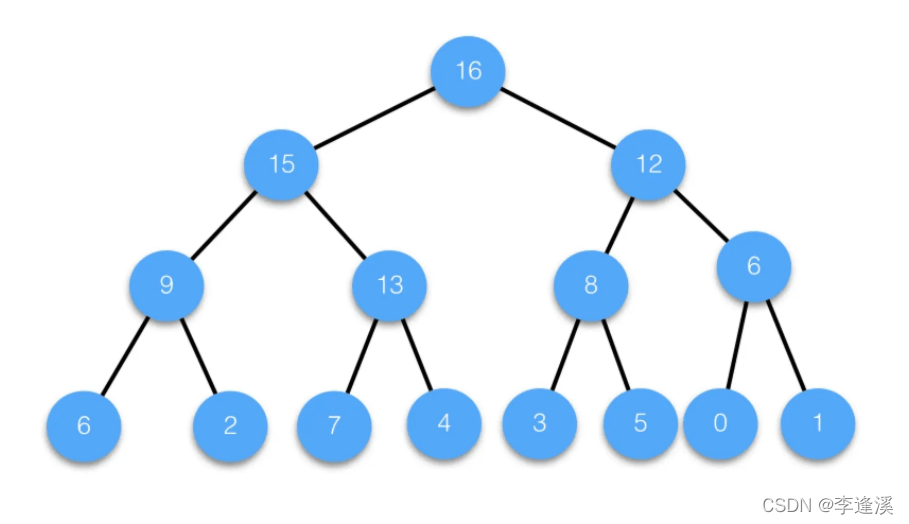﻿ C语言数据结构中堆排序的分析总结_C 语言_脚本之家
C 语言# C语言数据结构中堆排序的分析总结

• 向上调整
• 向下调整
• 堆排序

## 二、堆

### 2.1堆的介绍（三点）

1.物理结构是数组

2.逻辑结构是完全二叉树

3.大堆:所有的父亲节点都大于等于孩子节点，小堆：所有的父亲节点都小于等于孩子节点。

### 2.2向上调整```void AdjustUp(HPDataType* a, int child)
{
int parent = (child - 1) / 2;
while (child > 0)
{
if (a[child] > a[parent])//如果孩子大于父亲，则将它们交换。
{
Swap(&a[child], &a[parent]);
//迭代过程：
child = parent;
parent = (child - 1) / 2;
}
else
{
//如果孩子小于父亲，结束调整
break;
}
}
}```

### 2.3向下调整如图根为23，它的左右子树都是大堆，但整颗完全二叉树不是堆，通过向下调整可以使得整颗完全二叉树是堆。

```void AdjustDown(HPDataType* a, int size, int root)
{
int parent = root;
int child = parent * 2 + 1;//左孩子
while (child < size)
{
if (child + 1 < size && a[child] < a[child + 1])//如果左孩子小于右孩子，则选右孩子
{
//务必加上child+1，因为当child=size-1时，右孩子下标是size，对其接引用会越界访问。
child++;//右孩子的下标等于左孩子+1
}
if (a[child] > a[parent])//让较大的孩子与父亲比较，如果孩子大于父亲，则将它们交换。
{
Swap(&a[child], &a[parent]);
//迭代过程
parent = child;
child = parent * 2 + 1;
}
else
{
break;
}
}
}```

### 2.4建堆（两种方式）```for (int i = 1; i < n; i++)
{
}```

时间复杂度计算：

```//n代表数组元素个数，j的初始值代表最后一个元素的父亲下标
for (int j = (n - 1 - 1) / 2; j >= 0; j--)
{
}```T=2^(h-2)*1+2^(h-3)*2+2^(h-4)*3+.....+2^3*(h-4)+2^2*(h-3)+2^1*(h-2)+2^0*(h-1)

## 三、堆排序

```    int end = n - 1;//n代表数组元素的个数
while (end > 0)
{
Swap(&a, &a[end]);
end--;
}```

```void Swap(int* a, int* b)
{
int temp = *a;
*a = *b;
*b = temp;
}

{
int parent = (child - 1) / 2;
while (child > 0)
{
if (a[child] > a[parent])
{
Swap(&a[child], &a[parent]);
child = parent;
parent = (child - 1) / 2;
}
else
{
break;
}
}
}
void AdjustDown(int* a, int n, int root)
{
int child = 2 * root + 1;
while (child < n)
{
if (child + 1 < n && a[child] < a[child+1])
{
child++;
}
if (a[child] > a[root])
{
Swap(&a[child], &a[root]);
root = child;
child = 2 * root + 1;
}
else
{
break;
}
}
}

void HeapSort(int* a, int n)
{
//建大堆(向上调整)
//for (int i = 1; i < n; i++)
//{
//}

//建大堆(向下调整)
for (int j = (n - 1 - 1) / 2; j >= 0; j--)
{
}
//升序
int end = n - 1;
while (end > 0)
{
Swap(&a, &a[end]);
end--;
}
}
void printarr(int* a, int n)
{
for (int i = 0; i < n; i++)
{
printf("%d ", a[i]);
}
printf("\n");
}

int main()
{
int arr = { 9,2,4,8,6,3,5,1,10 };
int size = sizeof(arr) / sizeof(arr);
HeapSort(arr, size);
printarr(arr, size);
return 0;
}```